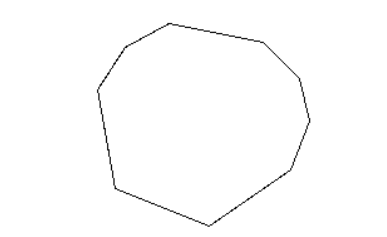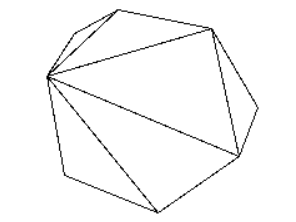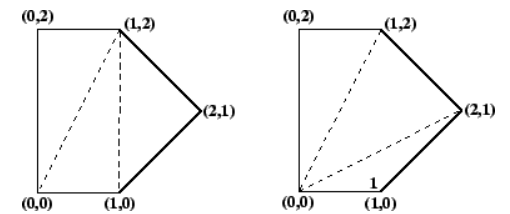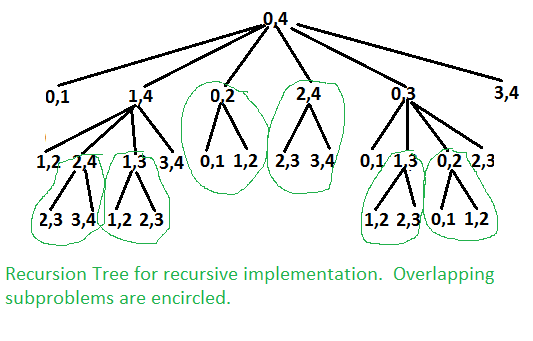×

Search anything:

# Minimum Cost for Triangulation of a Convex Polygon

#### Algorithms Dynamic Programming (DP)Get this book -> Problems on Array: For Interviews and Competitive Programming

Reading time: 30 minutes | Coding time: 10 minutes

A polygon is a two-dimensional closed shape defined by connections between points or vertices. A convex polygon has the following properties:

• It is simple, i.e., doesn't cross itself.
• Any line intersecting the polygon crosses the boundary at most twice.

For example, this is a convex polygon:We can represet a polygon with n+1 points as a sequence of vertices listed in counterclockwise order, i.e., P = <v0, v1, ... , vn>, has n+1 sides, <v0,v1>, <v1,v2>, ... , <vn-1,vn>. <vn,v0>.

A chord is a line segment connecting any two vertices. A chord splits the polygon into two smaller polygons. Note that a chord always divides a convex polygon into two convex polygons.

A triangulation of a polygon can be thought of as a set of chords that divide the polygon into triangles such that no two chords intersect (except possibly at a vertex). This is a triangulation of the same polygon:We are going consider following example in implementation.Two triangulations of the same convex pentagon.

An optimal triangulation is one that minimizes some cost function of the triangles. A common cost function is the sum of the lengths of the legs of the triangles that is:

``````cost (<vi, vj, vk>) = |vi,vj| + |vj, vk| + |vi,vk|
(where |a, b| is the Euclidean distance from point a to point b).
``````

We'll use this function for the discussion, although any function will work with the dynamic programming algorithm presented.

We would like to find, given a convex polygon and cost function over its vertices, the cost of an optimal triangulation of it. We would also like to get the structure of the triangulation itself as a list of triples of vertices.

This problem has a nice recursive substructure, a prerequisite for a dynamic programming algorithm. The idea is to divide the polygon into three parts:

• a single triangle
• the sub-polygon to the left
• the sub-polygon to the right.

We try all possible divisions like this until we find the one that minimizes the cost of the triangle plus the cost of the triangulation of the two sub-polygons. Where do we get the cost of triangulation of the two sub-polygons? Recursively, of course!

The base case of the recursion is a line segment (i.e., a polygon with zero area), which has cost 0.

Let's define a function based on this intuitive notion. Let t(i, j) be the cost of an optimal triangulation of the polygon <vi-1, vi, vi+1, ... , vj>. So

``````t(i, j) = MINIMUM cost for point i-1 to j
``````

Base case:

``````t(i, j) = 0, if i=j

mini <= k <= j-1 { t(i,k) + t(k+1,j) + cost (<vi-1, vk, vj>)} if i < j
``````

If we just have a line segment, that is, we're just looking at the "polygon" <vi-1, vj>, so i=j, then t(i, j) is just 0.

Otherwise, we let k go from i to j-1, looking at the sum of the costs of all triangles <vi-1, vk, vj> and all polygons <vi-1, ..., vk> and <vk+1, ..., vj> and finding the minimum.

``````for k from i to j-1
t(i, j) = MINIMUM{ t(i, j), t(i, k) + t(k+1, j) }
``````

Then t(1, n) is the cost of an optimal triangulation for the entire polygon.

``````t(1, n) is our answer
``````

In our above give example, triangulation on the left has a cost of 8 + 2√2 + 2√5 (approximately 15.30), the one on the right has a cost of 4 + 2√2 + 4√5 (approximately 15.77).

We just look at all possible triangles and leftover smaller polygons and pick the configuration that minimizes the cost.

First we will see the recursive function

Following is the pseudocode for naive recursive function.

``````Let Minimum Cost of triangulation of vertices from i to j be minCost(i, j)
If j <= i + 2 Then
minCost(i, j) = 0
Else
minCost(i, j) = Min { minCost(i, k) + minCost(k, j) + cost(i, k, j) }
Here k varies from 'i+1' to 'j-1'

Cost of a triangle formed by edges (i, j), (j, k) and (k, i) is
cost(i, j, k)  = dist(i, j) + dist(j, k) + dist(k, i)
``````

Following is C++ implementation of above naive recursive formula.

``````// Recursive implementation for minimum cost convex polygon triangulation
#include <iostream>
#include <cmath>
#define MAX 1000000.0
using namespace std;

// Structure of a point in 2D plane
struct Point
{
int x, y;
};

// Utility function to find minimum of two double values
double min(double x, double y)
{
return (x <= y)? x : y;
}

// A utility function to find distance between two points in a plane
double dist(Point p1, Point p2)
{
return sqrt((p1.x - p2.x)*(p1.x - p2.x) +
(p1.y - p2.y)*(p1.y - p2.y));
}

// A utility function to find cost of a triangle. The cost is considered
// as perimeter (sum of lengths of all edges) of the triangle
double cost(Point points[], int i, int j, int k)
{
Point p1 = points[i], p2 = points[j], p3 = points[k];
return dist(p1, p2) + dist(p2, p3) + dist(p3, p1);
}

// A recursive function to find minimum cost of polygon triangulation
// The polygon is represented by points[i..j].
double mTC(Point points[], int i, int j)
{
// There must be at least three points between i and j
// (including i and j)
if (j < i+2)
return 0;

// Initialize result as infinite
double res = MAX;

// Find minimum triangulation by considering all
for (int k=i+1; k<j; k++)
res = min(res, (mTC(points, i, k) + mTC(points, k, j) +
cost(points, i, k, j)));
return res;
}

// Driver program to test above functions
int main()
{
Point points[] = {{0, 0}, {1, 0}, {2, 1}, {1, 2}, {0, 2}};
int n = sizeof(points)/sizeof(points);
cout << mTC(points, 0, n-1);
return 0;
}
``````

Output:

``````15.3006
``````

The above problem is similar to Matrix Chain Multiplication. The following is recursion tree for mTC(points[], 0, 4).This situation is perfectly suited for dynamic programming. There are many redundant computations done. Each time we find a value for t(i, j), we can just stick that value into a two-dimensional array and never have to compute it again.

Here is the algorithm to compute the value of an optimal triangulation of a polygon given as an array V[0..n] of vertices. The array memo_t is an n by n array of real numbers initialized to -1. We must be careful to do arithmetic on vertex indices modulo n+1, to avoid going outside the array:

``````Triangulate (V)
weight = t (1, n)
print "the weight is" weight

t (i, j)
if i == j then return 0
if memo_t[i][j] != -1 then return memo_t[i][j]
min = infinity
for k in i..j-1 do
x = t (i, k) + t (k+1 (mod n+1), j)
+ cost (<v[i-1 (mod n+1)], v[k], v[j]>)
if x < min then min = x
end for
memo_t[i][j] = min
return min
``````

This takes O(n^2) storage because of the big array, and O(n^3) time since we have a function that does n things on n^2 array elements.

Implementation of the above pseudo code is given below.

``````// A Dynamic Programming based program to find minimum cost of convex
// polygon triangulation
#include <iostream>
#include <cmath>
#define MAX 1000000.0
using namespace std;

// Structure of a point in 2D plane
struct Point
{
int x, y;
};

// Utility function to find minimum of two double values
double min(double x, double y)
{
return (x <= y)? x : y;
}

// A utility function to find distance between two points in a plane
double dist(Point p1, Point p2)
{
return sqrt((p1.x - p2.x)*(p1.x - p2.x) +
(p1.y - p2.y)*(p1.y - p2.y));
}

// A utility function to find cost of a triangle. The cost is considered
// as perimeter (sum of lengths of all edges) of the triangle
double cost(Point points[], int i, int j, int k)
{
Point p1 = points[i], p2 = points[j], p3 = points[k];
return dist(p1, p2) + dist(p2, p3) + dist(p3, p1);
}

// A Dynamic programming based function to find minimum cost for convex
// polygon triangulation.
double mTCDP(Point points[], int n)
{
// There must be at least 3 points to form a triangle
if (n < 3)
return 0;

// table to store results of subproblems. table[i][j] stores cost of
// triangulation of points from i to j. The entry table[n-1] stores
// the final result.
double table[n][n];

// Fill table using above recursive formula. Note that the table
// is filled in diagonal fashion i.e., from diagonal elements to
// table[n-1] which is the result.
for (int gap = 0; gap < n; gap++)
{
for (int i = 0, j = gap; j < n; i++, j++)
{
if (j < i+2)
table[i][j] = 0.0;
else
{
table[i][j] = MAX;
for (int k = i+1; k < j; k++)
{
double val = table[i][k] + table[k][j] + cost(points,i,j,k);
if (table[i][j] > val)
table[i][j] = val;
}
}
}
}
return table[n-1];
}

// Driver program to test above functions
int main()
{
Point points[] = {{0, 0}, {1, 0}, {2, 1}, {1, 2}, {0, 2}};
int n = sizeof(points)/sizeof(points);
cout << mTCDP(points, n);
return 0;
}

``````

Output:

``````15.3006
``````#### Parth Maniyar

Competitive Programmer | Intern at OpenGenus | Bachelor of Technology (2017 to 2021) in Information Technology at Ahmedabad University

Minimum Cost for Triangulation of a Convex Polygon